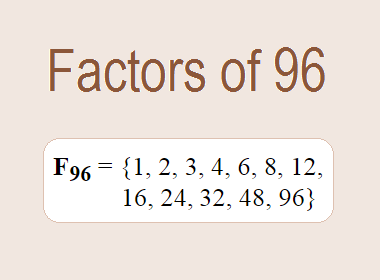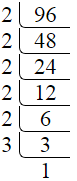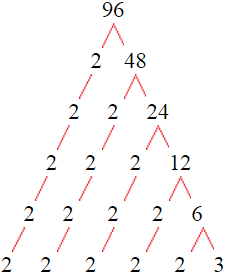# Factors of 96The factors of 96 are 1, 2, 3, 4, 6, 8, 12, 16, 24, 32, 48, and 96 i.e. F96 = {1, 2, 3, 4, 6, 8, 12, 16, 24, 32, 48, 96}. The factors of 96 are those numbers that can divide 96 without leaving a remainder.

We can check if these numbers are factors of 96 by dividing 96 by each of them. If the result is a whole number, then the number is a factor of 96. Let's do this for each of the numbers listed above:

·        1 is a factor of 96 because 96 divided by 1 is 96.

·        2 is a factor of 96 because 96 divided by 2 is 48.

·        3 is a factor of 96 because 96 divided by 3 is 32.

·        4 is a factor of 96 because 96 divided by 4 is 24.

·        6 is a factor of 96 because 96 divided by 6 is 16.

·        8 is a factor of 96 because 96 divided by 8 is 12.

·        12 is a factor of 96 because 96 divided by 12 is 8.

·        16 is a factor of 96 because 96 divided by 16 is 6.

·        24 is a factor of 96 because 96 divided by 24 is 4.

·        32 is a factor of 96 because 96 divided by 32 is 3.

·        48 is a factor of 96 because 96 divided by 48 is 2.

·        96 is a factor of 96 because 96 divided by 96 is 1.

## How to Find Factors of 96?

1 and the number itself are the factors of every number. So, 1 and 96 are two factors of 96. To find the other factors of 96, we can start by dividing 96 by the numbers between 1 and 96. If we divide 96 by 2, we get a remainder of 0. Therefore, 2 is a factor of 96. If we divide 96 by 3, we get a remainder of 0. Therefore, 3 is also a factor of 96.

Next, we can check if 4 is a factor of 96. If we divide 96 by 4, we get a remainder of 0. Therefore, 4 is also a factor of 96. If we divide 96 by 5, we get a remainder of 1. Therefore, 5 is not a factor of 96. We can continue this process for all the possible factors of 96.

Through this process, we can find that the factors of 96 are 1, 2, 3, 4, 6, 8, 12, 16, 24, 32, 48, and 96. These are the only numbers that can divide 96 without leaving a remainder.

********************

********************

## Properties of the Factors of 96

The factors of 96 have some interesting properties. One of the properties is that the sum of the factors of 96 is equal to 234. We can see this by adding all the factors of 96 together:

1 + 2 + 3 + 4 + 6 + 8 + 12 + 16 + 24 + 32 + 48 + 96 = 252

Another property of the factors of 96 is that they are all composite numbers except 1, 2, and 3.

## Applications of the Factors of 96

The factors of 96 have several applications in mathematics. One of the applications is in finding the highest common factor (HCF) of two or more numbers. The HCF is the largest factor that two or more numbers have in common. For example, to find the HCF of 96 and 120, we need to find the factors of both numbers and identify the largest factor they have in common. The factors of 96 are 1, 2, 3, 4, 6, 8, 12, 16, 24, 32, 48, and 96. The factors of 120 are 1, 2, 3, 5, 6, 8, 10, 12, 15, 20, 24, 30, 40, 60, and 120. The largest factor that they have in common is 24. Therefore, the HCF of 96 and 120 is 24.

Another application of the factors of 96 is in prime factorization. Prime factorization is the process of expressing a number as the product of its prime factors. The prime factors of 96 are 2, and 3, since these are the only prime numbers that can divide 96 without leaving a remainder. Therefore, we can express 96 as:

96 = 2 × 2 × 2 × 2 × 2 × 3

We can do prime factorization by division and factor tree method also. Here is the prime factorization of 96 by division method,96 = 2 × 2 × 2 × 2 × 2 × 3

Here is the prime factorization of 96 by the factor tree method,96 = 2 × 2 × 2 × 2 × 2 × 3

## Conclusion

The factors of 96 are the numbers that can divide 96 without leaving a remainder. The factors of 96 are 1, 2, 3, 4, 6, 8, 12, 16, 24, 32, 48, and 96. The factors of 96 have some interesting properties, such as having a sum of 252. The factors of 96 have several applications in mathematics, such as finding the highest common factor and prime factorization.